# AP Calculus AB Practice Test 36

### Test Information10 questions20 minutes

Calculator Disallowed

1.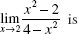2.3. If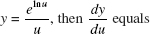4. Using the line tangent to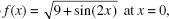an estimate of f (0.06) is

5. Air is escaping from a balloon at a rate of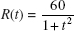cubic feet per minute, where t is measured in minutes. How much air, in cubic feet, escapes during the first minute?

6. If y = sin3 (1 - 2x), then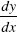is

7. If y = x2e1/x (x ≠ 0), then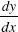is

8. A point moves along the curve y = x2 + 1 so that the x-coordinate is increasing at the constant rate of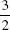units per second. The rate, in units per second, at which the distance from the originis changing when the point has coordinates (1, 2) is equal to

9.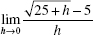10. The base of a solid is the first-quadrant region bounded by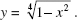Each cross section perpendicular to the x-axis is a square with one edge in the xy-plane. The volume of the solid is